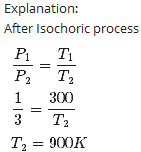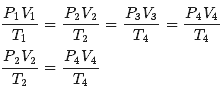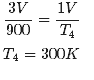Courses

# Test: Kinetic Theory (CBSE Level With Solutions)

## 30 Questions MCQ Test Physics Class 11 | Test: Kinetic Theory (CBSE Level With Solutions)

Description
This mock test of Test: Kinetic Theory (CBSE Level With Solutions) for Class 11 helps you for every Class 11 entrance exam. This contains 30 Multiple Choice Questions for Class 11 Test: Kinetic Theory (CBSE Level With Solutions) (mcq) to study with solutions a complete question bank. The solved questions answers in this Test: Kinetic Theory (CBSE Level With Solutions) quiz give you a good mix of easy questions and tough questions. Class 11 students definitely take this Test: Kinetic Theory (CBSE Level With Solutions) exercise for a better result in the exam. You can find other Test: Kinetic Theory (CBSE Level With Solutions) extra questions, long questions & short questions for Class 11 on EduRev as well by searching above.
QUESTION: 1

### Kinetic theory explains the behavior

Solution:

Explanation:

The theory for ideal gases makes the following assumptions

1. Gases consist of particles in constant, random motion. They continue in a straight line until they collide with something—usually each other or the walls of their container.

2. Particles are point masses with no volume. The particles are so small compared to the space between them, that we do not consider their size in ideal gases.

3. No molecular forces are at work. This means that there is no attraction or repulsion between the particles.

4. Gas pressure is due to the molecules colliding with the walls of the container. All of these collisions are perfectly elastic, meaning that there is no change in energy of either the particles or the wall upon collision. No energy is lost or gained from collisions.

5. The time it takes to collide is negligible compared with the time between collisions.

6. The kinetic energy of a gas is a measure of its Kelvin temperature. Individual gas molecules have different speeds, but the temperature and kinetic energy of the gas refer to the average of these speeds.

7. The average kinetic energy of a gas particle is directly proportional to the temperature. An increase in temperature increases the speed in which the gas molecules move.

8. All gases at a given temperature have the same average kinetic energy.

9. Lighter gas molecules move faster than heavier molecules.

QUESTION: 2

### The number of degrees of freedom a diatomic molecule is

Solution:

A diatomic molecule has 5 degrees of freedoms - 3 translational degrees each in one principal axis and 2 rotational degrees of freedom.

QUESTION: 3

### Three vessels of equal capacity have gases at the same temperature and pressure. The first vessel contains neon (monatomic), the second contains chlorine (diatomic), and the third contains uranium hexafluoride (polyatomic). The number of molecules

Solution:

Explanation:Avogadro's law states that, "equal volumes of all gases, at the same temperature and pressure, have the same number of molecules".

QUESTION: 4

A 14.5 kg mass, fastened to the end of a steel wire of unstretched length 1.0 m, is whirled in a vertical circle with an angular velocity of 2 rev/s at the bottom of the circle. The cross-sectional area of the wire is 0.065 cm2. Calculate the elongation of the wire when the mass is at the lowest point of its path.

Solution:

Mass, m = 14.5 kg
Length of the steel wire, l = 1.0 m
Angular velocity, ω = 2 rev/s = 2 × 2π rad/s = 12.56 rad/s
Cross-sectional area of the wire, a = 0.065 cm2 = 0.065 × 10-4 m2
Let Δl be the elongation of the wire when the mass is at the lowest point of its path.
When the mass is placed at the position of the vertical circle, the total force on the mass is:
F = mg + mlω2
= 14.5 × 9.8 + 14.5 × 1 × (12.56)2
= 2429.53 N
Young’s modulus = Strss / Strain
Y = (F/A) / (∆l/l)
∴ ∆l = Fl / AY
Young’s modulus for steel = 2 × 1011 Pa
∆l = 2429.53 × 1 / (0.065 × 10-4 × 2 × 1011)   =   1.87 × 10-3 m
Hence, the elongation of the wire is 1.87 × 10–3 m
Hence 1.87 × 10–3 m

QUESTION: 5

One mole of an ideal monatomic gas is at an initial temperature of 300 K. The gas undergoes an isovolumetric process, acquiring 500 J of energy by heat. It then undergoes an isobaric process, losing this same amount of energy by heat. Determine the new temperature of the gas

Solution:

Explanation:

for monoatomic  gas CV = 1.5R, CP =  2.5R

At const volume,

Q = 500 J

Q=nCVΔT

500=1×1.5×8.31(T1−300)

T1=340K

At const pressure Q = 500 J

Q=nCPΔT

500=1×2.5×8.31(340−T2)

T2=316K

QUESTION: 6

Kinetic theory

Solution:

Explanation:The kinetic theory describes a gas as a large number of submicroscopic particles (atoms or molecules), all of which are in constant rapid motion that has randomness arising from their many collisions with each other and with the walls of the container.It will applicable only for gases

QUESTION: 7

The number of degrees of freedom a monatomic molecule is

Solution:

Explanation:Degrees of freedom of a system refers to the possible independent motions a system can have.Monoatomic gas molecule can have 3 independent motion and hence have 3 degrees of freedom (all are translational).

QUESTION: 8

Calculate the change in internal energy of 3.00 mol of helium gas when its temperature is increased by 2.00 K.

Solution:

Explanation:

Helium is a monoatomic gas.(CV = 1.5R)

change in internal  energy

ΔU=nCVΔT=3×1.5×8.31×2=75J

QUESTION: 9

One mole of an ideal monatomic gas is at an initial temperature of 300 K. The gas undergoes an isovolumetric process, acquiring 500 J of energy by heat. It then undergoes an isobaric process, losing this same amount of energy by heat. Determine the work done on the gas.

Solution:

Explanation:

At const volume,

Q = 500 J

Q=nCPΔT

500=1×2.5×8.31ΔT

ΔT=24.06

W=nRΔT=1×8.31×24.06=200J

QUESTION: 10

Kinetic theory relates measurable properties.

Solution:

Explanation:Kinetic theory explains the behaviour of gases based on the idea that the gas consists of rapidly moving atoms or molecules. It also relates measurable properties of gases such as viscosity, conduction and diffusion with molecular parameters, yielding estimates of molecular sizes and masses.

QUESTION: 11

Mean free path is the

Solution:

Explanation:the mean free path is the average distance traveled by a moving particle (such as an atom, a molecule, a photon) between successive impacts (collisions), which modify its direction or energy or other particle properties.

QUESTION: 12

According to Atomic Hypothesis:

Solution:

Explanation:atomic hypothesis that all things are made of atoms—little particles that move around in perpetual motion, attracting each other when they are a little distance apart, but repelling upon being squeezed into one another.

QUESTION: 13

1 mole of a monoatomic gas is mixed with 3 moles of a diatomic gas. What is the molecular specific heat of the mixture at constant volume?

Solution:

Explanation:

for monoatomic gas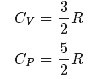from conservation of energy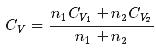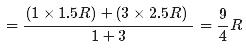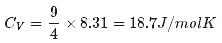QUESTION: 14

Solution:

Explanation:Avogadro's law states that, "equal volumes of all gases, at the same temperature and pressure, have the same number of molecules"For a given mass of an ideal gas, the volume and amount (moles) of the gas are directly proportional if the temperature and pressure are constant.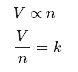QUESTION: 15

One mole of hydrogen gas is heated at constant pressure from 300 K to 420 K. Calculate the energy transferred by heat to the gas

Solution:

Explanation:

Hydrogen is a diatomic gas. (C= 3.5R)

energy transferred by heat to the gas

Q=nCPΔT=1×3.5×8.31×120=3490J=3.49KJ

QUESTION: 16

In which case are the atoms relatively rigidly fixed?

Solution:

Explanation:

Atoms in a gas are well separated with no regular arrangement. Atoms vibrate and move freely at high speeds

Atoms in a liquid are close together with no regular arrangement. Atoms vibrate, move about, and slide past each other.

Atoms in a solid are tightly packed, usually in a regular pattern. Atoms vibrate (jiggle) but generally do not move from place to place.

QUESTION: 17

One mole of hydrogen gas is heated at constant pressure from 300 K to 420 K. Calculate the increase in its internal energy.

Solution:

Explanation:

Hydrogen is a diatomic gas. (C= 2.5R)

Change in internal energy

ΔnCVΔ= 1 x 2.5 x 8.31 x 120 = 2493= 2.49KJ

QUESTION: 18

Two moles of an ideal gas (γ=1.4) expands slowly and adiabatically from a pressure of 5.00 atm and a volume of 12.0 L to a final volume of 30.0 L. What is the final pressure of the gas?

Solution: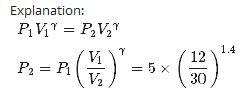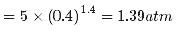QUESTION: 19

The average distance a molecule can travel without colliding is called the

Solution:

Explanation:the mean free path is the average distance traveled by a moving particle (such as an atom, a molecule, a photon) between successive impacts (collisions), which modify its direction or energy or other particle properties

QUESTION: 20

According to Atomic Hypothesis: little particles of atom

Solution:

Explanation:At room temperature (=300K) the noble gases are all in the gas phase, they are banging around and colliding into one another like little pool balls. At this temperature, when the atoms collide they appear to elastically bounce off of one another, but this bounce is actually a result of atomic repulsion. The atoms are traveling so fast and they approach each other so quickly that their momentum 'squeezes' them together until the atomic repulsion pushes them back apart.

QUESTION: 21

In dynamic equilibrium, molecules collide and change their speeds during the collision

Solution:

Explanation:All collisions between molecules among themselves or between molecules and the walls are elastic. So that total kinetic energy and total momentum is conserved. Also the average properties of gas are remains constant.

QUESTION: 22

One mole of hydrogen gas is heated at constant pressure from 300 K to 420 K. Calculate the work done by the gas.

Solution:

Explanation:

In  isobaric process

W=nR(TFTI)=1×8.31×(420−300)=0.98kJ

QUESTION: 23

The average kinetic energy of a molecule

Solution:

Explanation: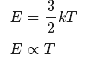QUESTION: 24

Figure shows plot of PV/T versus P for 1.00x10-3 kg of oxygen gas at two different temperatures. What is the value of PV/T where the curves meet on the y-axis?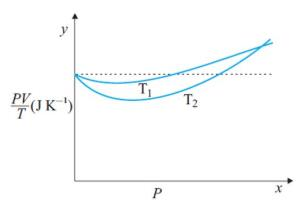Solution:

PV=nRT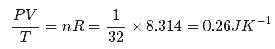Hence the value of PV/T where the curves meet on the y-axis is 0.26 jK-1

QUESTION: 25

Nine particles have speeds of 5.00, 8.00, 12.0, 12.0, 12.0, 14.0, 14.0, 17.0, and 20.0 m/s. find the particles’ average speed.

Solution: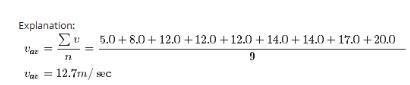QUESTION: 26

What is the root-mean-square speed of helium atoms in a balloon of diameter 30.0 cm at 20.00C and 1.00 atm?

Solution: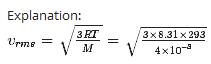= 1351m/sec = 1.35 Km/sec

QUESTION: 27

For a rope of yield strength Sy loaded in tension with weight Mg the minimum area A of the rope should be

Solution:

F = YA (∆l/l)
F = Mg, Y = Sy, A = A using this we get
So, Mg ≤ Sy x A => A ≥ Mg/Sy

QUESTION: 28

Estimate the total number of air molecules (inclusive of oxygen, nitrogen, water vapor and other constituents) in a room of capacity 25.0 m3 at a temperature of 270C and 1 atm pressure.

Solution: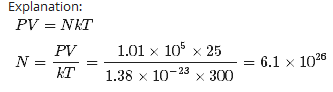QUESTION: 29

In a 30.0-s interval, 500 hailstones strike a glass window with an area of 0.600 m2 at an angle of 45.0° to the window surface. Each hailstone has a mass of 5.00 g and a speed of 8.00 m/s. If the collisions are elastic, what is the pressure on the window?

Solution: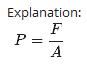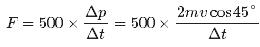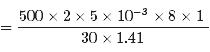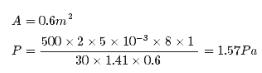QUESTION: 30

Four liters of a diatomic ideal gas ( λ =1.4) confined to a cylinder is subject to a closed cycle. Initially, the gas is at 1.00 atm and at 300 K. First, its pressure is tripled under constant volume. Then, it expands adiabatically to its original pressure. Finally, the gas is compressed isobarically to its original volume. Find the temperature at the end of the cycle

Solution: